# 0x00 二叉树

A full binary tree (sometimes referred to as a proper or plane binary tree) is a tree in which every node has either 0 or 2 children.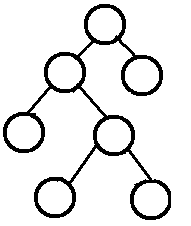A perfect binary tree is a binary tree in which all interior nodes have two children and all leaves have the same depth or same level.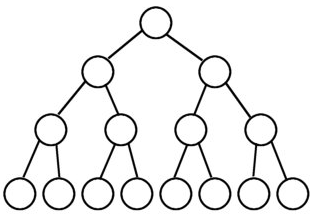1. 非空二叉树第 $i$ 层上最多有 $2^{i-1}$ 个结点

2. 高度为 $h$ 的二叉树至多有 $2^h-1$ 个结点

3. $i$ 个结点所在的层次（深度）为 $\lfloor\log_2{i}\rfloor+1$

4. 具有 $N$ 个结点的完全二叉树的高度为 $\lceil\log_2{(N+1)}\rceil$

5. 对于任意二叉树，度为0的结点记为 $N_0$ ，度为2的结点记为 $N_2$ ，则必有 $N_0=N_2+1$ ，即度为0的结点总比度为2的结点多一个

6. 一棵具有 $N$ 个结点的树，所有结点的度数之和为 $N-1$

7. 度为n的结点，第i层最多有 $n^{i-1}$ 个结点

$\lfloor x\rfloor$ 为向下取整符号，结果为不大于x的最大整数，例如 $\lfloor2.9\rfloor=2$

$\lceil x\rceil$ 为向上取整符号，结果为不小于x的最小整数，例如 $\lceil2.1\rceil=3$

# 0x01 二叉树的遍历

## 0x00 先序遍历

ABDCEGFHI

class Solution {public:    std::vector<int> preorderTraversal(TreeNode* root) {        std::vector<int> ret;        if (root)        {            ret.emplace_back(root->val);            std::vector<int> left = preorderTraversal(root->left);            ret.insert(ret.end(), left.begin(), left.end());            std::vector<int> right = preorderTraversal(root->right);            ret.insert(ret.end(), right.begin(), right.end());        }        return ret;    }};

## 0x01 中序遍历

BDAGECHFI

class Solution {public:    std::vector<int> inorderTraversal(TreeNode* root) {        std::vector<int> ret;        if (root)        {            std::vector<int> left = inorderTraversal(root->left);            ret.insert(ret.end(), left.begin(), left.end());            ret.emplace_back(root->val);            std::vector<int> right = inorderTraversal(root->right);            ret.insert(ret.end(), right.begin(), right.end());        }        return ret;    }};

## 0x02 后序遍历

DBGEHIFCA

class Solution {public:    std::vector<int> postorderTraversal(TreeNode* root) {        std::vector<int> ret;        if (root)        {            std::vector<int> left = postorderTraversal(root->left);            ret.insert(ret.end(), left.begin(), left.end());            std::vector<int> right =                                    postorderTraversal(root->right);            ret.insert(ret.end(), right.begin(), right.end());            ret.emplace_back(root->val);        }        return ret;    }};

class Solution {public:    std::vector<int> postorderTraversal(TreeNode* root) {        std::vector<int> ret;        if (root)        {            std::stack<TreeNode*> stk;            stk.push(root);            while (!stk.empty())            {                TreeNode *dummy = stk.top();                stk.pop();                if (dummy->left)                {                    stk.push(dummy->left);                }                if (dummy->right)                {                    stk.push(dummy->right);                }                ret.emplace_back(dummy->val);            }            std::reverse(ret.begin(), ret.end());        }        return ret;    }};

## 0x03 层序遍历

ABCDEFGHI

class Solution {​    void levelOrder(std::vector<std::vector<int>> &res, std::vector<TreeNode*> current)    {        std::vector<int> nums;        std::vector<TreeNode*> next;        for (TreeNode* node : current)        {            if (node)            {                nums.emplace_back(node->val);                next.emplace_back(node->left);                next.emplace_back(node->right);            }        }        if (nums.size())        {            res.emplace_back(nums);        }        if (next.size())        {            levelOrder(res, next);        }    }public:    std::vector<std::vector<int>> levelOrder(TreeNode* root) {        std::vector<TreeNode*> current;        current.emplace_back(root);        std::vector<std::vector<int>> res;        levelOrder(res, current);        return res;    }};

# 0x02 二叉树的建立

## 0x00 什么样的遍历顺序组合可以独一地确立一颗二叉树

  1 /  2

      1    |     1    /      |       \  2        |         2树1        |         树2

$\frac{1}{N+1}C_{2N}^{N}$

## 0x01 通过先序遍历和中序遍历建立二叉树

class Solution {​    TreeNode * buildTree(std::vector<int>& preorder, int ps, std::vector<int>& inorder, int is, int ie)    {        if (ps >= preorder.size() || is >= ie)        {            return nullptr;        }        TreeNode *root = new TreeNode(preorder[ps]);        int i = is;        for (; i < ie; ++i)        {            if (inorder[i] == preorder[ps])            {                break;            }        }        root->left = buildTree(preorder, ps + 1, inorder, is, i);        root->right = buildTree(preorder, ps + i - is + 1, inorder, i + 1, ie);        return root;    }public:    TreeNode * buildTree(std::vector<int>& preorder, std::vector<int>& inorder) {        return buildTree(preorder, 0, inorder, 0, inorder.size());    }};

ABDCEGFHI

BDAGECHFI

class Solution {​    std::unordered_map<int, int> iMap;​    TreeNode * buildTree(std::vector<int>& preorder, int ps, std::vector<int>& inorder, int is, int ie)    {        if (ps >= preorder.size() || is >= ie)        {            return nullptr;        }        TreeNode *root = new TreeNode(preorder[ps]);        int i = iMap[preorder[ps]];        root->left = buildTree(preorder, ps + 1, inorder, is, i);        root->right = buildTree(preorder, ps + i - is + 1, inorder, i + 1, ie);        return root;    }public:    TreeNode * buildTree(std::vector<int>& preorder, std::vector<int>& inorder) {        for (int i = 0; i < inorder.size(); ++i)        {            iMap[inorder[i]] = i;        }        return buildTree(preorder, 0, inorder, 0, inorder.size());    }};

## 0x02 通过中序遍历和后序遍历建立二叉树

class Solution {​    std::unordered_map<int, int> iMap;​    TreeNode * buildTree(std::vector<int>& inorder, int is, int ie, std::vector<int>& postorder, int ps, int pe)    {        if (is >= ie || ps >= pe)        {            return nullptr;        }        TreeNode *root = new TreeNode(postorder[pe - 1]);        int lsize = iMap[postorder[pe - 1]] - is;        root->left = buildTree(inorder, is, is + lsize, postorder, ps, ps + lsize);        root->right = buildTree(inorder, iMap[postorder[pe - 1]] + 1, ie, postorder, ps + lsize, pe - 1);        return root;    }public:    TreeNode * buildTree(std::vector<int>& inorder, std::vector<int>& postorder) {        for (int i = 0; i < inorder.size(); ++i)        {            iMap[inorder[i]] = i;        }        return buildTree(inorder, 0, inorder.size(), postorder, 0, postorder.size());    }};

# 0x03 线索二叉树

## 0x00 基本概念

struct TreeNode {    int val;          // 数据域    TreeNode *left;   // 左孩子    TreeNode *right;  // 右孩子    int ltag;         // 左标识域    int rtag;         // 右标识域}

• ltag

• 0：left指针指向结点的左孩子

• 1：left指针指向结点的前驱

• rtag

• 0：right指针指向结点的右孩子

• 1：right指针指向结点的后继

N个结点的线索二叉树含有N+1个线索。

## 0x01 建立中序线索二叉树

struct TreeNode {    int val;    bool ltag; // false: left child, true: previous    bool rtag; // false: right child, true: succeed    TreeNode *left;    TreeNode *right;    TreeNode(int x) : val(x), ltag(false), rtag(false), left(nullptr), right(nullptr) {}};​class Solution {​    void inThreading(TreeNode *root, TreeNode *&pre)    {        if (root)        {            inThreading(root->left, pre); // 线索化左子树            if (!root->left)            {                root->left = pre;                root->ltag = true;            }            if (pre && !pre->right)            {                pre->right = root;                pre->rtag = true;            }            pre = root;            inThreading(root->right, pre); // 线索化右子树        }    }public:    TreeNode * convertToThreadedTreeWithInorderTraversal(TreeNode *root)    {        TreeNode *pre = nullptr;        inThreading(root, pre);        return root;    }};

## 0x02 建立后序线索二叉树

// 二叉树结点的存储结构参考建立中序线索二叉树的代码​class Solution {​    void inThreading(TreeNode *root, TreeNode *&pre)    {        if (root)        {            inThreading(root->left, pre);            inThreading(root->right, pre);            if (!root->left)            {                root->left = pre;                root->ltag = true;            }            if (pre && !pre->right)            {                pre->right = root;                pre->rtag = true;            }            pre = root;        }    }public:    TreeNode * convertToThreadedTreeWithPostorderTraversal(TreeNode *root)    {        TreeNode *pre = nullptr;        inThreading(root, pre);        return root;    }};

# 0x04 树、森林与二叉树之间的相互转换

## 0x00 树转化为二叉树

1. 树中所有带有相同双亲的兄弟结点之间加一条连线

2. 对树中不是双亲结点的第一个孩子的结点，只保留新添加的该结点与左兄弟结点之间的连线，删去该节点与双亲结点之间的连线

## 0x01 二叉树转化为树

1. 若某结点是其双亲结点的左孩子，则把该结点的右孩子，右孩子的右孩子等等等等都与这些结点的双亲结点连起来

2. 删除原二叉树中所有双亲结点与右孩子结点的连线

## 0x02 二叉树转化为森林

1. 从根节点开始，若右孩子存在，则把与右孩子结点的连线删除

2. 再查看分离后的二叉树，若其根节点的右孩子存在，则连线删除

3. 循环往复直到所有这些根节点与右孩子的连线都删除为止

4. 然后将分离的二叉树全部转化为树

## 0x03 森林转化为二叉树

1. 将森林中的每棵树变为二叉树

2. 因为转换所得的二叉树的根结点的右子树均为空，故可将各二叉树的根结点视为兄弟从左至右连在一起，就形成了一棵二叉树

# 0x05 二叉排序树（又名二叉搜索树或二叉查找树）

• 若左子树非空，则子树上所有结点的关键字值均于根节点的关键字值

• 若右子树非空，则子树上所有结点的关键字值均于根节点的关键字值

• 左右子树本身分别也是一颗二叉排序树

## 0x00 二叉排序树的插入

class Solution {​public:    TreeNode * insertIntoBST(TreeNode* root, int val) {        if (!root)        {            return new TreeNode(val);        }        if (root->val < val)        {            root->right = insertIntoBST(root->right, val);        }        else if (root->val > val)        {            root->left = insertIntoBST(root->left, val);        }        return root;    }};

class Solution {​public:    TreeNode * insertIntoBST(TreeNode* root, int val) {        std::stack<TreeNode*> stk;        TreeNode **target{ &root };        TreeNode *dummy{ root };        while (dummy)        {            if (dummy->val < val)            {                stk.push(dummy->right);                target = &dummy->right;            }            else if (dummy->val > val)            {                stk.push(dummy->left);                target = &dummy->left;            }            else            {                return root;            }            dummy = stk.top();            stk.pop();        }        *target = new TreeNode(val);        return root;    }};

class Solution {​public:    TreeNode * insertIntoBST(TreeNode* root, int val) {        TreeNode *next;        TreeNode **target{ &root };        TreeNode *dummy{ root };        while (dummy)        {            if (dummy->val < val)            {                next = dummy->right;                target = &dummy->right;            }            else if (dummy->val > val)            {                next = dummy->left;                target = &dummy->left;            }            else            {                return root;            }            dummy = next;        }        *target = new TreeNode(val);        return root;    }};

## 0x01 二叉排序树的删除

• 如果被删除的结点为叶子结点，那将其直接删除，并不会破坏二叉树的性质

• 如果被删除的结点仅有左子树或者右子树，则让其子树直接代替被删除的结点

• 如果被删除的结点同时存在左子树和右子树，用被删除节点的直接前驱或者直接后继来替换当前节点，调整直接前驱或者直接后继的位置，如图：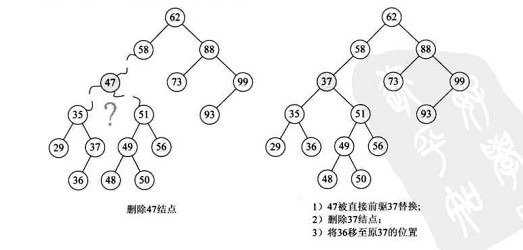在上图中，我们要删除47结点，这个结点既有左孩子又有右孩子，我们首先看其中序遍历的序列：

29, 35, 36, 37, 47, 48, 49, 50, 51, 56, 58, 62, 73, 88, 93, 99

要删除的结点47的直接前驱为37，所以我们把37拿上去换掉被删除的47，这样就转换成了第一或第二种状况，然后再依第一第二种状况处理即可。

root = [5,3,6,2,4,null,7]key = 3​    5   / \  3   6 / \   \2   4   7​Given key to delete is 3. So we find the node with value 3 and delete it.​One valid answer is [5,4,6,2,null,null,7], shown in the following BST.  // 使用其直接后继取代被删除的结点​    5   / \  4   6 /     \2       7​Another valid answer is [5,2,6,null,4,null,7].// 使用其直接前驱取代被删除的结点​    5   / \  2   6   \   \    4   7// 两种答案均可

class Solution {​public:    TreeNode * deleteNode(TreeNode* root, int key) {        if (!root)        {            return nullptr;        }        if (root->val > key)        {            root->left = deleteNode(root->left, key);        }        else if (root->val < key)        {            root->right = deleteNode(root->right, key);        }        else        {            if (root->left && root->right)            {                TreeNode *next = root->right;                while (next->left)                {                    next = next->left;                }                std::swap(root->val, next->val);                root->right = deleteNode(root->right, key);            }            else if (root->left || root->right)            {                TreeNode *ret =                               root->left ? root->left : root->right;                delete root;                return ret;            }            else            {                delete root;                return nullptr;            }        }        return root;    }};

• 其同时具有左右孩子，那寻找其中序遍历的直接后继，然后交换当前结点与其直接后继的值，将其进一步转换为下面的两种情况，继续递归

• 其仅具有一个孩子，那就用其仅具有的这个孩子来代替这个结点即可

• 其为叶子结点，直接将其删除，不对二叉排序树的性质产生任何的影响

## 0x02 二叉排序树的查找

class Solution {public:    TreeNode * searchBST(TreeNode* root, int val) {        if (root == nullptr)        {            return nullptr;        }        if (root->val > val)        {            return searchBST(root->left, val);        }        else if (root->val < val)        {            return searchBST(root->right, val);        }        return root;    }};

## 0x03 二叉排序树的判定

class Solution {​    void inorder(TreeNode *root, std::vector<int>& result)    {        if (!root)        {            return;        }        inorder(root->left, result);        result.push_back(root->val);        inorder(root->right, result);    }public:    bool isValidBST(TreeNode* root) {        if (!root)        {            return true;        }        std::vector<int> dummy;        inorder(root, dummy);        for (int i = 1; i < dummy.size(); ++i)        {            if (dummy[i - 1] >= dummy[i])            {                return false;            }        }        return true;    }};

    1   /   1

class Solution {​    bool isValidBST(TreeNode* root, long min, long max)    {        if (!root)        {            return true;        }        if (root->val <= min || root->val >= max)        {            return false;        }        return isValidBST(root->left, min, root->val) &&            isValidBST(root->right, root->val, max);    }public:    bool isValidBST(TreeNode* root) {        if (!root)        {            return true;        }        return isValidBST(root, LONG_MIN, LONG_MAX);    }};

# 0x06 平衡二叉树

## 0x00 基本概念

• 其左子树和右子树均为平衡二叉树

• 左子树和右子树高度差的绝对值不超过1

## 0x01 平衡二叉树的判定

class Solution {​    int depth(TreeNode *root)    {        if (!root)        {            return 0;        }        return std::max(depth(root->left) + 1, depth(root->right) + 1);    }public:    bool isBalanced(TreeNode* root) {        return !root ||             std::abs(depth(root->left) - depth(root->right)) < 2 &&            isBalanced(root->left) && isBalanced(root->right);    }};

## 0x02 平衡二叉树的插入

• 首先按照二叉排序树的插入流程来插入结点w

• 从w开始，向上遍历并找到第一个导致此树不平衡的结点z，将从结点z到结点w路径上的z的孩子记为y，将此路径上的z的孙子结点记为x

• 对以z为根节点的子树进行旋转使整个树重新趋于平衡，有如下4种可能的情况

• y是z的左孩子且x是y的左孩子，执行LL平衡旋转（右单旋转或Left Left Case），如下 ：

T1, T2, T3 and T4 are subtrees.         z                                      y         / \                                   /   \       y   T4      Right Rotate (z)          x      z      / \          - - - - - - - - ->      /  \    /  \      x   T3                               T1  T2  T3  T4    / \  T1   T2
• y是z的左孩子且x是y的右孩子，执行LR平衡旋转（先左后右双旋转或Left Right Case），如下 ：

     z                               z     / \                            /   \   y   T4  Left Rotate (y)        x    T4   / \      - - - - - - - - ->    /  \ T1   x                          y    T3    / \                        / \  T2   T3                    T1   T2                      x                    /   \ Right Rotate(z)    y      z- - - - - - - ->  / \    / \                 T1 T2  T3  T4
• y是z的右孩子并且x是y的右孩子，执行RR平衡旋转（左单旋转或Right Right Case），如下：

  z                                y /  \                            /   \ T1   y     Left Rotate(z)       z      x    /  \   - - - - - - - ->    / \    / \   T2   x                     T1  T2 T3  T4       / \     T3  T4
• y是z的右孩子并且x是y的左孩子，执行RL平衡旋转（先右后左双旋转或Right Left Case），如下：

   z                           z   / \                         / \        T1   y   Right Rotate (y)    T1  x    / \  - - - - - - - - ->     /  \      x   T4                      T2   y            / \                              /  \T2   T3                           T3   T4                       x                      /  \ Left Rotate(z)      z      y- - - - - - - ->   / \    / \                 T1  T2  T3  T4

GeeksforGeeks有一个视频详细讲述了上述的旋转过程，链接如下：

class Solution {​    int height(TreeNode *root)    {        if (!root)        {            return 0;        }        return std::max(height(root->left), height(root->right))+1;    }​    /*     *        y                           x     *       / \     Right Rotation      /  \     *      x   T3   – – – – – – – >    T1   y      *     / \       < - - - - - - -        / \     *    T1  T2     Left Rotation         T2  T3     */    TreeNode * leftRotate(TreeNode *root)    {        TreeNode *x = root;        TreeNode *y = x->right;        TreeNode *T2 = y->left;        y->left = x;        x->right = T2;        return y;    }​    TreeNode * rightRotate(TreeNode *root)    {        TreeNode *y = root;        TreeNode *x = root->left;        TreeNode *T2 = x->right;        x->right = y;        y->left = T2;        return x;    }​    TreeNode * insertToAVL(TreeNode *root, int num)    {        // Perform standard BST insert        if (!root)        {            return new TreeNode(num);        }        if (root->val < num)        {            root->right = insertToAVL(root->right, num);        }        else if (root->val > num)        {            root->left = insertToAVL(root->left, num);        }        else        {            return root;        }        //         int leftHight = height(root->left);        int rightHeight = height(root->right);        int balance = leftHight - rightHeight;        /*         *                  z               *                 / \            *  root->left->  y   T4         *               / \             *              x   T3           *             / \         *           T1   T2         *  balance > 1,          *  the height of left subtree is greater than         *  right tree more than 1,          *  and the new node's value is lesser than y,          *  so it will be inserted at the left subtree of y,         *  performs right rotation to rebalance the tree         */        if (balance > 1 && num < root->left->val)        {            return rightRotate(root);        }        /*         *        z           *       / \          *      y   T4          *     / \         *   T1   x         *       / \          *     T2   T3               *  the new node's value is greater than y,          *  so it will be inserted at the right subtree of y,         *  performs left rotation of left subtree         *  and then right rotation of the root         */        if (balance > 1 && num > root->left->val)        {            root->left = leftRotate(root->left);            return rightRotate(root);        }        /*         *      z         *     / \         *   T1   y  <- root->right         *       / \          *      x   T4               *     / \         *   T2   T3         *  balance < -1 means that         *  the height of right subtree is greater than          *  left subtree more than 1,         *  and the new node's value is lesser than y         *  performs right rotation of right subtree         *  and then left rotation of the root         */        if (balance < -1 && num < root->right->val)        {            root->right = rightRotate(root->right);            return leftRotate(root);        }        /*        *     z        *    /  \        *   T1   y        *       /  \        *      T2   x        *          / \        *        T3  T4        *  balance < -1 means that        *  the height of right subtree is greater than         *  left subtree more than 1,        *  and the new node's value is greater than y        *  performs left rotation of the root        */        if (balance < -1 && num > root->right->val)        {            return leftRotate(root);        }        //         return root;    }public:    TreeNode * sortedArrayToBST(std::vector<int>& nums) {        if (nums.size() == 0)        {            return nullptr;        }        TreeNode *root = new TreeNode(nums);        for (int i = 1; i < nums.size(); ++i)        {            root = insertToAVL(root, nums[i]);        }        return root;    }};

class Solution {​    TreeNode * sortedArrayToBST(std::vector<int>& nums, int left, int right)    {        if (left > right)        {            return nullptr;        }        int mid = left + (right - left) / 2;        TreeNode *root = new TreeNode(nums[mid]);        root->left = sortedArrayToBST(nums, left, mid - 1);        root->right = sortedArrayToBST(nums, mid + 1, right);        return root;    }public:    TreeNode * sortedArrayToBST(std::vector<int>& nums) {        return sortedArrayToBST(nums, 0, nums.size() - 1);    }};

class Solution {    TreeNode * sortedListToBST(ListNode *begin, ListNode *end)    {        if (!begin || begin == end)        {            return nullptr;        }        if (!begin->next || begin->next == end)        {            return new TreeNode(begin->val);        }        ListNode *slow = begin->next;        ListNode *fast = begin->next->next;        while (fast && fast != end && fast->next && fast->next != end)        {            slow = slow->next;            fast = fast->next->next;        }        TreeNode *root = new TreeNode(slow->val);        root->left = sortedListToBST(begin, slow);        root->right = sortedListToBST(slow->next, end);        return root;    }public:    TreeNode * sortedListToBST(ListNode* head) {        return sortedListToBST(head, nullptr);    }};

class Solution {public:    TreeNode* sortedListToBST(ListNode* head) {        if (!head) return nullptr;        if (!head->next) return new TreeNode(head->val);        ListNode *dummy = new ListNode(-1);        ListNode *slow = dummy, *fast =dummy;        dummy->next = head;        while ( fast->next && fast->next->next ) {            slow = slow->next;            fast = fast->next->next;        }        ListNode *center = slow->next;        slow->next = nullptr;        fast = center->next;        TreeNode *cur = new TreeNode(center->val);        if (head != center) cur->left = sortedListToBST(head);        cur->right = sortedListToBST(fast);        return cur;    }};

# 0x07 B树和B+树

## 0x00 B树基本概念

B树（B-tree）又称为多路平衡查找树，B树中所有结点的孩子结点数的最大值称为B数的阶（order），通常用m表示，一棵m阶B树（B tree of order m或m-order B tree）通常具有如下性质：

• 树中每个结点至多有m棵子树，即至多有m-1个关键字

• 若根节点不是终端结点，则至少含有2棵子树

• 除根节点外的所有非叶节点至少有 $\lceil \frac{m}{2} \rceil$ 棵子树，即至少含有 $\lceil \frac{m}{2} \rceil-1$ 个关键字

• 结点中关键字的个数n符合关系 $\lceil \frac{m}{2} \rceil-1 \leqslant n \leqslant m-1$

• 所有叶结点都出现在同一层次上，并且不带信息（这些结点实际上是不存在的，指向这些结点的指针为空）

| * | 25 | * | 30 | * | 33 | * | 37 | * |

## 0x01 B树的高度

B树中的大部分操作所需要的磁盘存取次数与B树的高度成正比。

• 关键字的个数n应满足 $n \leqslant m^h-1$ ，高度h则有 $h \geqslant \log_m{(n+1)}$

• 若让每个结点中关键字的个数达到最少，此时，容纳相同多元素的B树的高度可达到最大，由B树的定义，第一层至少有1个顶点，第二层至少有2个顶点，此后除根节点外的每个非终端结点至少有 $\lceil \frac{m}{2} \rceil$ 棵子树，则第三层至少有 $2 \times \lceil \frac{m}{2} \rceil$ 个结点，以此类推，第h+1层（叶子结点层）至少有 $2 \times \lceil \frac{m}{2} \rceil^{h-1}$ 个结点

• B树的高度范围$\log_m{(n+1)} \leqslant h \leqslant \log_{\lceil \frac{m}{2} \rceil}{(\frac{n+1}{2})}+1$

## 0x02 B树的查找

B树的查找类似于二叉排序树的查找，只不过是每个结点变成了有多个关键字的有序表，所以每个结点上所做的并不像二叉排序树一样是两路分支决定，而是多路分支决定。故其查找过程包含两个基本操作

• 首先在B树中找结点

• 然后在结点中找关键字

B树往往被存储在磁盘中，故前一个操作往往在磁盘中进行，当找到目标结点时，先将结点中的信息读入内存，然后采用顺序查找法或二分查找查找等于目标值的关键字。如果查找到叶节点（没有存放任何信息的结点）则说明查找失败。

## 0x03 B树的插入

• 定位：利用B树的查找算法，找出插入该关键字的最底层中某个非叶结点

• 插入：在B树中，每个非失败结点的关键字的个数都在区间 $[\lceil \frac{m}{2} \rceil-1, m-1]$ 内，当插入后的结点关键字个树仍在上述区间内（小于m），那么可以直接插入，否则，必需对结点进行分裂：

• 取一个新结点，将插入K后的原结点从中间位置将其中的关键字分为两部分

• 左半部分保持在原结点中

• 右半部分包含的关键字放入新结点中

• 中间位置的结点（ $\lceil \frac{m}{2} \rceil$ ）插入原结点的父节点中

• 若此时父节点的关键字个数也超过了上限，则继续执行上述的分裂操作，直到这个过程传递到根节点，导致B树的高度加一（注意看下图insert(7)的过程）

B树中插入关键字一定是插入在最底层的某个非叶结点内

## 0x04 B树的删除

B树的删除操作类似于插入操作，同样需保证删除之后的结点中的关键字个数大于等于 $\lceil \frac{m}{2} \rceil-1$ ，因此涉及到合并问题。B树的删除有如下几种情况：

• 当被删除的关键字所在的结点的关键字个数大于 $\lceil \frac{m}{2} \rceil-1$ ，那么删除之后的树，仍然满足B树的定义，所以可以直接将其删除，如下图中删除D的过程。

• 当被删除的关键字所在结点删除前关键字个数恰好等于 $\lceil \frac{m}{2} \rceil-1$ ，且与此相邻的右（左）兄弟结点的关键字个数大于等于 $\lceil \frac{m}{2} \rceil$ ，此时兄弟够借，则需要调整该节点右（左）兄弟结点以及其双亲结点，也就是从旁边借一个节点过来调整以达到新的平衡，如下图中删除关键字F：

• 当被删除的关键字所在结点删除前关键字个数恰好等于 $\lceil \frac{m}{2} \rceil-1$ ，且此时与此结点相邻的左右兄弟结点的关键字个数也等于 $\lceil \frac{m}{2} \rceil-1$ ，此时左右兄弟不够借了，那么就需要将关键字删除后与右（左）兄弟结点及双亲结点中的关键字进行合并，如下图删除关键字B的过程：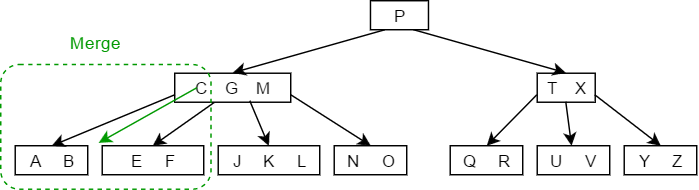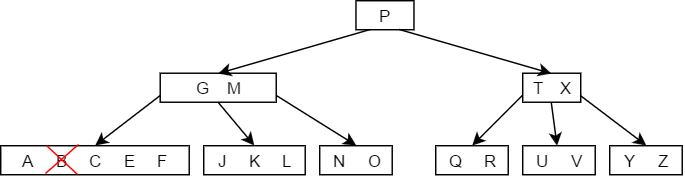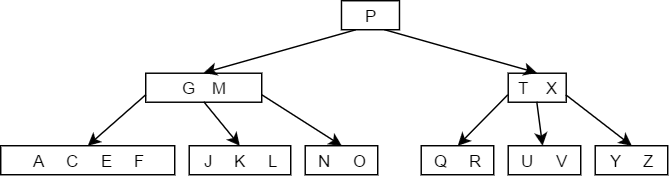在合并的过程中，双亲结点中的关键字个数会减少，若其双亲结点时根节点并且关键字个数减少至0，则直接将根节点删除，合并后的新结点成为根，如果双亲结点不是根节点，且关键字的个数减少至 $\lceil \frac{m}{2} \rceil - 2$ ，那么又要与它自己的兄弟结点进行调整或合并操作，直到符合B树的要求为止。

• 如果小于K的子树中关键字的个数大于 $\lceil \frac{m}{2} \rceil-1$ ，那么直接用K的前驱值来代替K即可，如下图删除结点M的过程：

• 如果大于K的子树中关键字的个数大于 $\lceil \frac{m}{2} \rceil-1$ ，则找出K的后继值，用其后继值来代替K，如下图中删除结点G的过程：